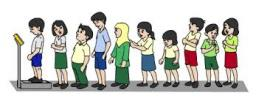# Gymnasiums 5550

Half of the 9th-grade students want to study at secondary technical schools, a quarter at vocational schools, a sixth at gymnasiums, and 3 students do not wish to continue their studies. How many pupils are there in the class?

n =  36

### Step-by-step explanation:

n/2 + n/4 + n/6 + 3 = n

n = 36

n = 36/1 = 36

n = 36

Our simple equation calculator calculates it.Did you find an error or inaccuracy? Feel free to write us. Thank you!

Tips for related online calculators
Need help calculating sum, simplifying, or multiplying fractions? Try our fraction calculator.
Do you have a linear equation or system of equations and looking for its solution? Or do you have a quadratic equation?